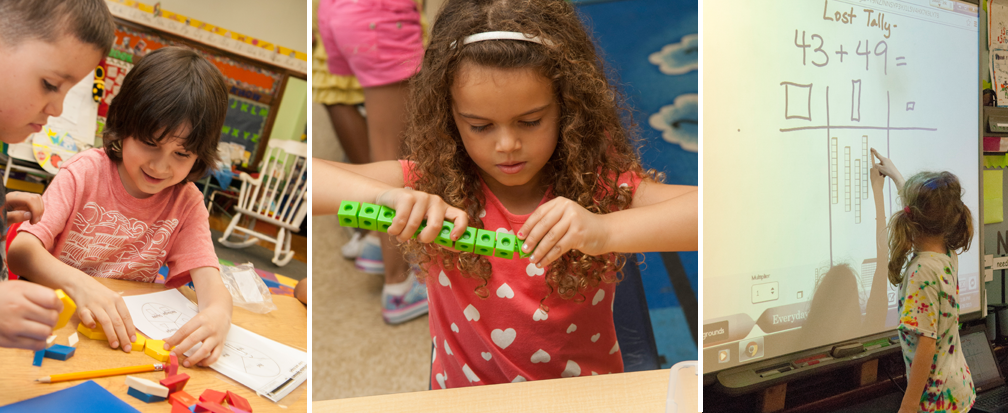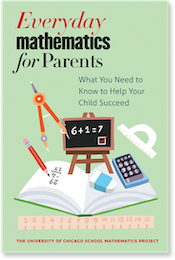## Games by Grade and Unit

Below are a number of resources for parents to further supplement lessons with online games to play at home.

The links below may provide students with an opportunity for practice. These websites are not connected to CEMSE or to Everyday Mathematics and our posting them does not constitute an endorsement.

### Kindergarten

Numeration
 Bunny Count Connect the Dots One False Move Snakes and Ladders Wash Line
Measurement
 Taller or Shorter Measure It Compare and Order
Geometry
 McGraw Hill Unit 5 Rats! Catch the Shapes Match Shapes to Symbols Matching Shapes Geoboard Shapes
Operations
Patterns
 Odd Ball Connect the Dots One False Move Pattern Mania Build your Own!
Money
 Match Coins Spending Spree

Unit 1 Routines
 Number Line Bounce Guess the Number One False Move Number Stories Bunny Count
Unit 2 Number Uses
 False Move Bunny Count Time to hours Pennies and Nickels
Unit 3 Patterns and Counting
 Guess the Number Connect the Numbers One False Move Number Cracker
Unit 4 Measurement/Facts
Unit 5 Place Value
 Baseball Math for one Mathcar Tic Tac Toe
Unit 6 Fact Power
 Mathcar Tic Tac Toe Geoboard Function box
Unit 7 Geometry/Attributes
 Attribute Blocks Fold the Shapes
Unit 8 Money/Fractions
 Change Maker Spending Spree Identifying Fractions
Unit 9 Place Value/Fractions
 Go Figure Test the Toad Guess the Number Can You Fill It? Identifying Fractions
Unit 10 Review

Unit 1 Numbers and Routines
 Learn to Count money Mend the Number Square Number Cracker Pattern Mania Guess the Number
 Baseball Math for Two Mathcar Tic Tac Toe Test the Toad Baseball Math for One Go Figure
Unit 3 Place Value, Time & Money
 Telling Time Game Counting Change What Time is It? Graph your data Change Maker
 Number Line Attribute Sort Measure It!
Unit 5 2D and 3D Shapes
 What's My Attribute Rule? Interactive Geoboard Arrays Polygons Tangrams
Unit 6 Whole Number Operations
Unit 7 Patterns and rules
 Pattern Generator Number Cracker Guess the Number Make Your Own Patternﾠ(100s chart) Pattern Blocks Design
Unit 8 Fractions
 What is a Fraction? Geoboards Interactive Fraction Bars Fresh Baked Fractions
Unit 9 Measurement
 Measure It! Perimeter of Rectangle Parallelogram Shape Surveyor
Unit 10 Place Value and Decimals
 Change Game Geoboards Interactive Arrays Base 10 Blocks (1,000s)
Unit 11 Whole Number Operation
 Baseball Math for One Baseball Math for Two Mathcar Tic Tac Toe
Unit 12 Review
 Baseball Math for One Baseball Math for Two Mathcar Tic Tac Toe

Unit 1 Routines
 Guess the Number Tally chart Bar graph Cookie Dough Number Sense
 Operation Order Mathcar Tic Tac Toe Baseball Math for One Seashell Rounding to 10s
Unit 3 Measure and Area
 Measure It! Shape Surveyor Interactive Geoboard - make your own! Interactive site to figure perimeter Interactive site to figure area
Unit 4 Multiplication & Division
 The Product Game Geoboards Interactive Arrays Baseball Math for one Baseball Math for two
 Mathcar Tic Tac Toe Multiplication Practice
Unit 5 Place value
 Base 10 blocks(1,000s) One False Move AAA Math Place Value 6 digits Decimals with Base 10
Unit 6 Geometry
 Isometric Drawing Tools Solids and Their Properties Reflection Symmetry
Unit 7 Multiplication/ Division
 The Product Game Baseball Math for One Mathcar Tic Tac Toe
Unit 8 Fractions
 Fresh Baked Fractions Fraction Tool Game Fraction Model Equivalent Fractions
Unit 9 Multiplication/ Division
 Factor Game The Product Game Grid Game Line Jumper Lattice Multiplication
Unit 10 Measurement
 Build a Box - Volume Animal Weigh In Data Handling - mean, median, mode Train Race Planet Hop
Unit 11 Probability

Unit 1 Name/Construct Geometric Figures
 Isometric Drawing Tools Pool Table Solids and Their Properties
Unit 2 Organizing Data
 Cookie Dough One False Move Seashell Rounding AAAMath Place Value AAAMath Place Value - 6 digits
 Train Race Baseball Math for Oneﾠ
Unit 3 Multiplication and Division
 Baseball Math for One Baseball Math for Two Mathcar Tic Tac Toe Operation Order Lattice Multiplication
Unit 4 Decimals
 AAAMath - decimals Decimal Place Value Adding Money Interactive Thousandths decimals Measure It!
Unit 5 Estimation
 Multiplying tens Rounding Off AAAMath Place Value
Unit 6 Division
 What's the Point Find Your Longitude Mathcar Tic Tac Toe Baseball Math for One
Unit 7 Fractions
 Fraction Tool Game Fresh Baked Fractions Soccer Shootout Fish Tank Saloon Snap
Unit 8 Area & Perimeter
 Interactive site to Figure A and P Area of Rectangle Area of Parallelogram Shape Surveyor Area of triangle
Unit 9 Percents
 Fraction Tool Game Saloon Snap Penguin Waiter
Unit 10 Reflection & Symmetry
 Bathroom Tiles Line Jumper Sort according to symmetry
Unit 11 Solids & Weights
 Build a Box - volume Isometric Drawing Tools Line Jumper Animal Weigh In
Unit 12 Rates

Unit 1 Number Theory
 The Product Game The Factor Game Grid Game
Unit 2 Estimation and Computation
 Baseball Math for One One False Move AAAMath Place Value - 6 digits
Unit 3 Geometry Explorations
 Solids and Their Properties Bathroom Tiles Isometric Drawing Tools
Unit 4 Division
 Baseball Math for One Baseball Math for Two Mathcar Tic Tac Toe
Unit 5 Fraction, Decimal & Percent
 Soccer Shootout Fresh Baked Fractions Interactive Fraction bars Penguin Waiter Rounding Off
 Saloon Snap Pie Graph
Unit 6 Fractions
 Mean, Median & Mode Stem and Leaf Plotter Soccer Shootout Fresh Baked Fractions
Unit 7 Exponents, Negative Numbers
 Powers of Tenvideo clip Basket Math Guess a Number Line Jumper
Unit 8 Fractions and Ratios
 Soccer Shootout Fraction Pie Game Fraction, Percent, Decimal Bars All About Ratios
Unit 9 Coordinates, Area, Volume
 Planet Hop Isometric Drawing Tools Shape Surveyor Build a Box - Volume
Unit 10 Algebra Concepts
 Shape Pan Balance Guess a number Circumference Area of circles Algebra Planet Blaster
Unit 11 Volume
 Illuminations Solids Isometric Drawing Tools Volume Capacity and Weight Volume and Surface AreaThe University of Chicago School Mathematics Project

University of Chicago Press